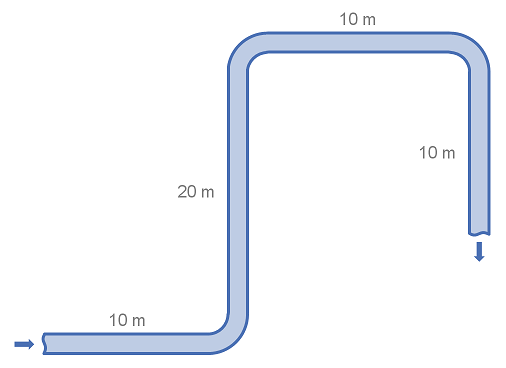# Summary

Fittings such as elbows, tees, valves and reducers represent a significant component of the pressure loss in most pipe systems. This article details the calculation of pressure losses through pipe fittings and some minor equipment using the 2K method.

# Definitions

 : Internal diameter of pipe (Inches) : Resistance Coefficient : Resistance Coefficient for fitting at : Resistance Coefficient for large fitting at : Reynolds number

# Introduction

The 2K method allows the user to characterise the pressure loss through fittings in a pipe. As the name suggests, two K coefficients are used to characterise the fitting, which when combined with the flow conditions and pipe diameter may be used to calculate the K-value (excess head), which is in turn used to calculate the head or pressure loss through the fitting via the excess head method.

The 2K Method is more accurate than the Equivalent Length method, or Excess Head (K-value) method as it applies an additional constant to improve characterisation of the fitting pressure drop with variation of the fluid Reynolds Number.

There is a further extension of the 2K method called the 3K method, which adds a third K coefficient and is reported to be more accurate than the 2K method, particularly for very large fittings.

For alternative methods of characterising pressure loss from fittings and a discussion of which method is most appropriate please refer to the summary of methods for estimating pressure loss from fittings.

# Calculation of K value using 2K Values

The 2K method is an extension of the Excess Head (K method) which attempts to take into account variations in pressure drop through a fitting caused by the size of the fitting and the fluid’s Reynolds number (particularly in lower Reynolds numbers).

## Formula for Calculating Excess Head Coefficients or K Values from 2K Values

The formula for calculating the excess head from the 2K values, Reynolds Number and pipe internal diameter is given below. Note that the pipe internal diameter is in inches.

From the K-value head loss through the fitting may be calculated.

## Values of K1 and K∞ for Various Fitting Types

Below is a list K-values for common fittings.

FittingTypeK1K
90° Elbow CurvedThreaded, SR (R/D = 1)8000.4
Flanged/Welded, SR (R/D = 1)8000.25
All Types, LR (R/D = 1.5)8000.2
90° Elbow Mitered
R/D 1.5
1 Weld (90° Angle)10001.15
2 Weld (45° Angle)8000.35
3 Weld (30° Angle)8000.3
4 Weld (22.5° Angle)8000.27
5 Weld (18° Angle)8000.25
45° ElbowAll Types, SR (R/D = 1)5000.2
All Types LR (R/D = 1.5)5000.15
45° Elbow Mitered1 Weld (45° Angle)5000.25
2 Welds (45° Angle)5000.15
180°Screwed, SR (R/D = 1)10000.6
Flanged/Welded, SR (R/D = 1)10000.35
All Types, LR (R/D = 1.5)10000.3
Tee, used as elbowScrewed, SR (R/D = 1)5000.7
Screwed, LR8000.4
Flanged/Welded, SR (R/D = 1)8000.8
Stub-in-tpye Branch10001
Tee, Run ThroughScrewed2000.1
Flanged/Welded1500.05
Stub-in-type Branch1000
Valves, Gate/Ball/PlugFull Line Size, Beta = 13000.1
Reduced Trim, Beta = 0.95000.15
Reduced Trim, Beta = 0.810000.25
ValvesGlobe, Standard15004
Globe, Angle10002
Diaphragm, dam type10002
Butterfly8000.25
Check ValvesLift200010
Swing15001.5
Tiliting-disk10000.5

# Example Calculation of Pressure Loss using 2K-values

This example demonstrates how to use the 2K method to calculate the head loss through simple pipe and fitting arrangement. The example uses water in system of standard weight carbon steel pipe. Note that for this example we consider a flat system, with no elevation changes.## System details

 Pipe Size : DN100 (4") Pipe Diameter : 102.3 mm Pipe Length : 50 m Fittings : 3 x 90° long radius (R/D = 1.5) elbows Fluid Velocity : 3 m/s Fluid Density : 1000 kg/m3 Reynolds Number : 306,900 Friction Factor : 0.018 Gravitational Acceleration : 9.81 m/s2

## Calculation

Using the table of fittings and 2K-values above we find that the excess head or K-value for the 90° long radius elbow.

With this we can calculate the head loss for a single elbow.

Using the equation for head loss in pipe, we can calculate the loss through the straight piping:

The total head loss for the system is the addition of the head loss from the pipe and the fittings.

In the above example we first calculated the head loss for a single fitting and then multiplied by the number of fittings. It is also correct to add or multiply the K-values of fittings and then covert to a pressure or head loss. This is useful when there are many fittings of several types. However the 2K values must be converted to single K values for each fitting type individually.

Article Tags

# Subscribe to our mailing list

 Email Name